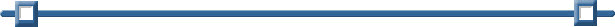Solving Equations The graphing calculator can be easily used to solve and/or check algebraic equations.
 Example 1:   Solve for x:   4x + 1 = 9 Method 1: Solve using nSolve From a Calculator page: MENU - #4 Calculations - #1 Numerical Solve (or use the Catalog) Enter the equation, followed by a comma and the name of the variable, which in this case is x. Hit ENTER. Answer:   x = 2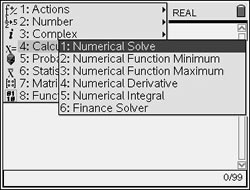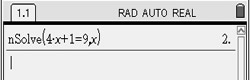Method 2: Solve graphically Enter the left side of the equation as f1(x). Enter right side of the equation as f2(x). Check to see if you need to adjust your window to see where the two graphs intersect. Find the point of intersection (the answer). (MENU - #6 Points & Lines - #3 Intersection Points) Click on both graphs. The ordered pair (2,9) is the intersection with the x-value being 2. Answer:   x = 2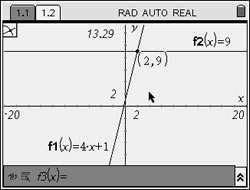Example 2:   Solve for x:   2x + 4 = 5x - 2 nSolve: x = 2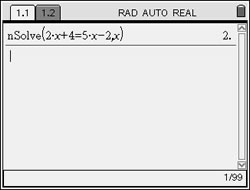Graphically: x = 2Example 3:   Solve for x: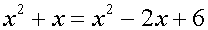nSolve: x = 2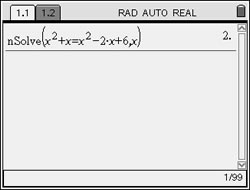Graphically: x = 2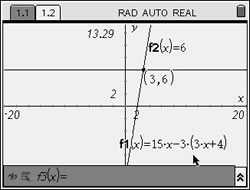Example 4:.   Solve for x:   15x – 3(3x + 4) = 6.  Choose the correct value for x. (1) 1       (2)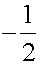(3) 3       (4)nSolve: Choice 3: x = 3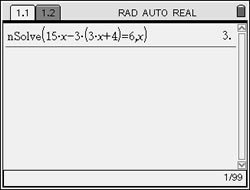Graphically: Choice 3: x = 3See "Quadratic Equations" for how to use nSolve with equations with more than one solution.